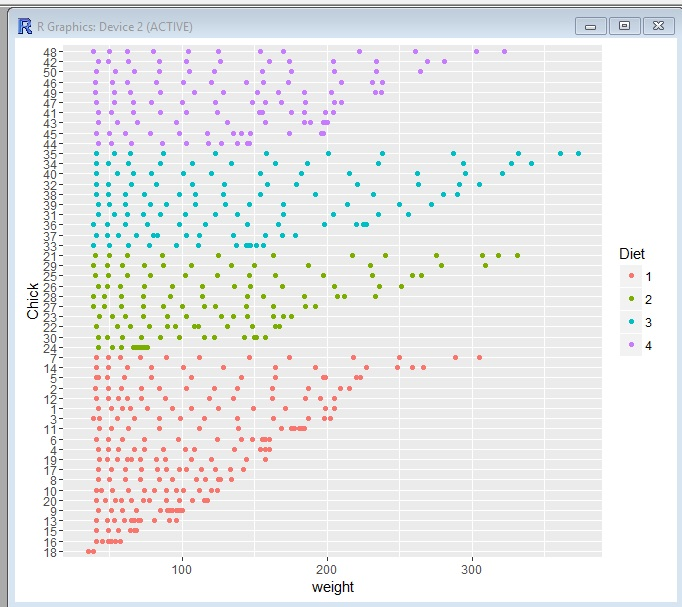# R: ggplot using facets

In this example I am going to teach you to use facets to add another level to your ggplot data visualizations. In this example we will be working with the ChickWeight data set from R Datasets

First, let’s check out the data by running head()

`head(ChickWeight)`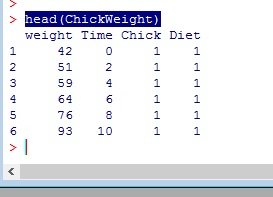We have 4 columns in our data, weight, Time, Chick, and Diet.

Now, let’s build our ggplot model. Below, we are setting our model to a variable “p1”. We then set ChickWeight as our data set and the weight column to our x axis. Remember, nothing will happen when running the line below.

`p1 <- ggplot(data=ChickWeight, aes(x=weight))`

Now lets graph our plot as a histogram – we will set our binwidth to 10 – if any of this confusing you, go back and check out my earlier lessons on ggplot and ggplot histograms

`p1 + geom_histogram(binwidth = 10)`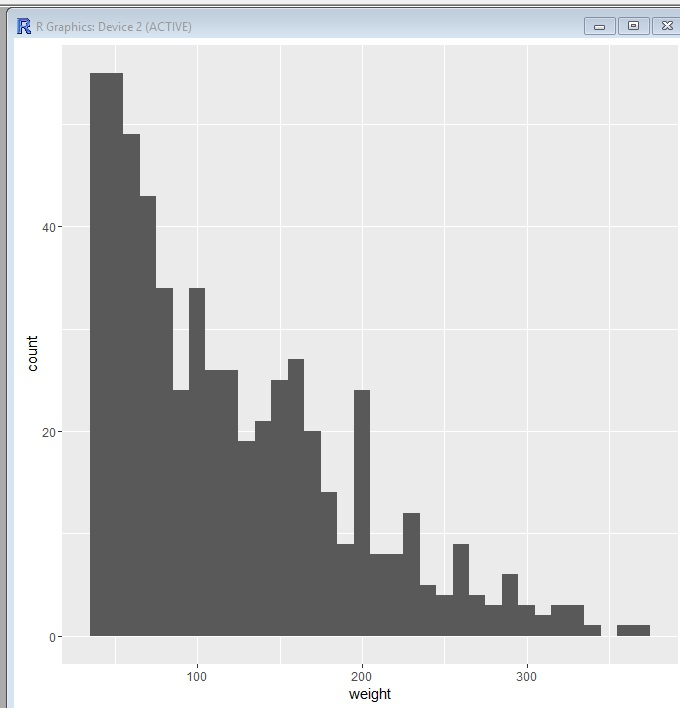Let’s now add some color. I am going to set our “fill” to be Diet

`p1 + geom_histogram(binwidth = 10, aes(fill=Diet))`Now, using facets, I am going to take it one step further and separate this into 4 histograms, one for each diet.

` p1 + geom_histogram(binwidth = 10, aes(fill=Diet)) + facet_grid(Diet~.)`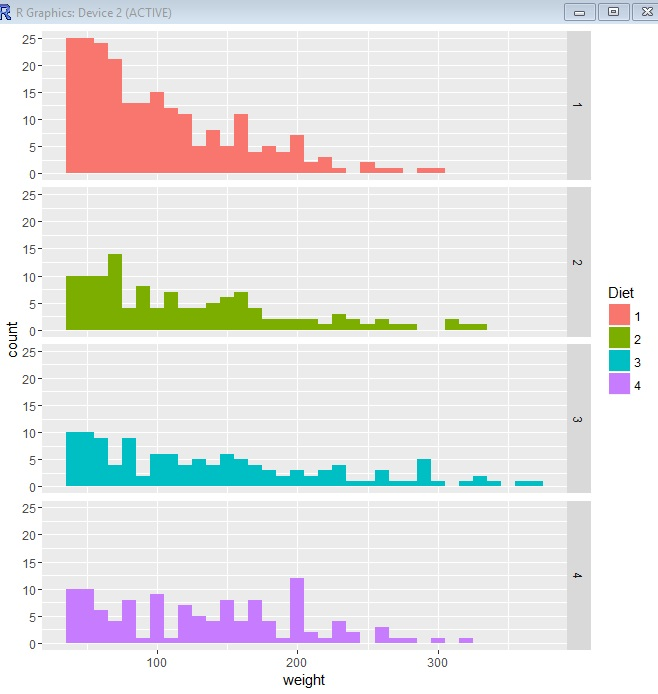## The Code

```#-- Look at data

#-- build ggplot model
p1 <- ggplot(data=ChickWeight, aes(x=weight))

#--plot ggplot as histogram
p1 + geom_histogram(binwidth = 10)

#-- set fill color to Diet
p1 + geom_histogram(binwidth = 10, aes(fill=Diet))

#-- create four histograms - one for each Diet
p1 + geom_histogram(binwidth = 10, aes(fill=Diet)) + facet_grid(Diet~.)```

# R: ggplot – Histograms

Let’s make a Histogram using ggplot

First step, import the ggplot2 library

`library(ggplot2)`

Now let’s look at our data. In this example I am using chickwts from R Data

```head(chickwts)

str(chickwts)```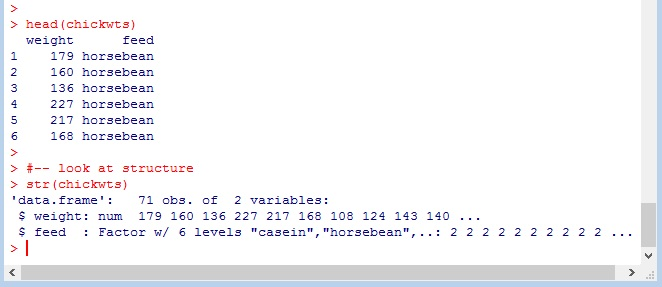As you can see above, this data contains 2 columns weight ( numeric) and feed(Factor with 6 levels)

## Histogram

In a histogram, we don’t need to worry about assigning a Y axis, the Y axis is the frequency count of our X variable.

Let’s set our data and x axis in ggplot. Let’s also assign it to variable.

`pl <- ggplot(data=chickwts, aes(x=weight))`

Now we can use the assigned variable in conjunction with our geom()

`pl + geom_histogram(binwidth=10)`

In our geom, I set a binwidth. This tells us how wide to make our bars. Setting binwidth to 10 makes each bar 10 units wide.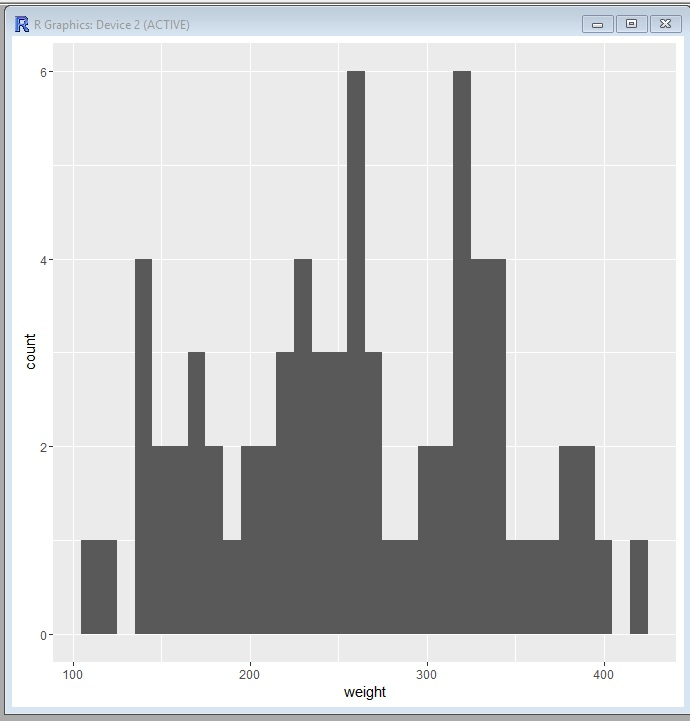Let’s give it some color

`pl + geom_histogram(binwidth=10, fill="blue")`Now, let’s set our colors based on our 2nd column “feed”. Note how a legend is automatically generated.

`pl + geom_histogram(binwidth=10, aes(fill=feed))`For the finishing touch, let’s add some black bordering around our boxes.

`pl + geom_histogram(binwidth=10, aes(fill=feed), color="black")`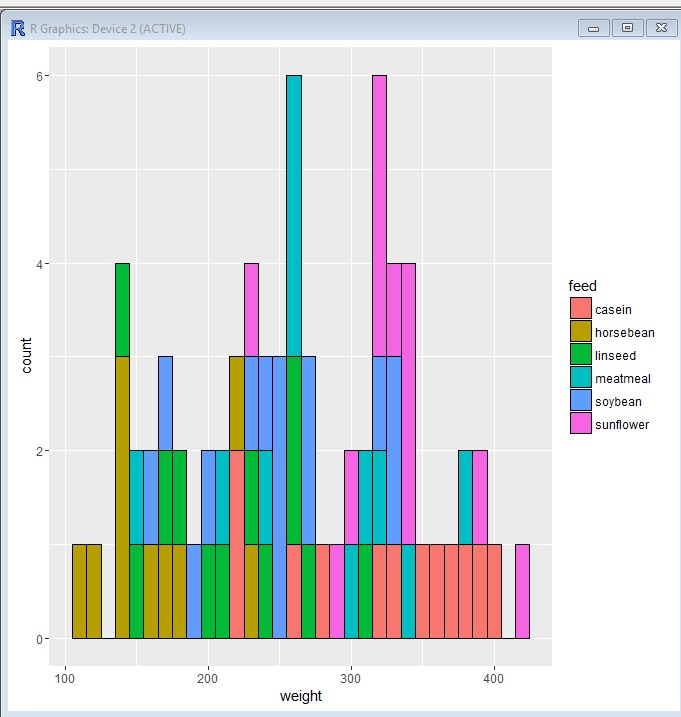## The Code

```library(ggplot2)

#-- look at structure
str(chickwts)

#set data and x axis value
pl <- ggplot(data=chickwts, aes(x=weight))

#create histogram, binwidth 10
pl + geom_histogram(binwidth=10)

#change bars to blue
pl + geom_histogram(binwidth=10, fill="blue")

#set color of bars by feed column values
pl + geom_histogram(binwidth=10, aes(fill=feed))

pl + geom_histogram(binwidth=10, aes(fill=feed), color="black")```

# R: Intro to ggplot()

ggplot() is a powerful graphing tool in R. While it is more complex to use than qplot(), its added complexity comes with advantages.

Don’t worry about the complexity, we are going to step into it slowly.

Let’s start by getting a data set. I am going to choose airquality from the R data sets package. You can find a list of data sets from that package here: Link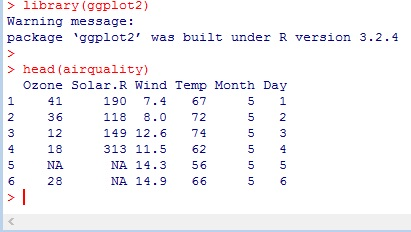We are going to manipulate some of this data, so first let us set the dataframe to a new variable.

Next, take a look at the structure – str() – we have 6 variables and 153 rows. The first thing I notice is that Month is an int – I don’t want that. I would rather have Month be a factor      (a categorical variable)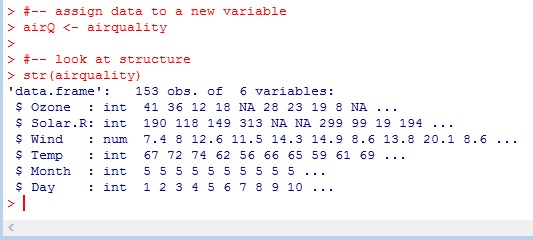Use factor() to set Month to a factor. Now look at str() readout again. There are 5 levels (months) represented in our data set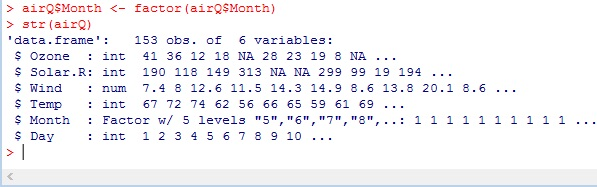## ggplot()

here is the basic syntax – ggplot(data, aes(x value, y value))+geom_[type of plot]

`ggplot(data = airQ,  aes(x = Wind, y = Temp)) + geom_point()`Now let’s add some color to the chart. Inside aes() – which stands for aesthetics – we are going to add color = Month

` ggplot(data = airQ, aes(x = Wind, y = Temp, color = Month)) + geom_point()`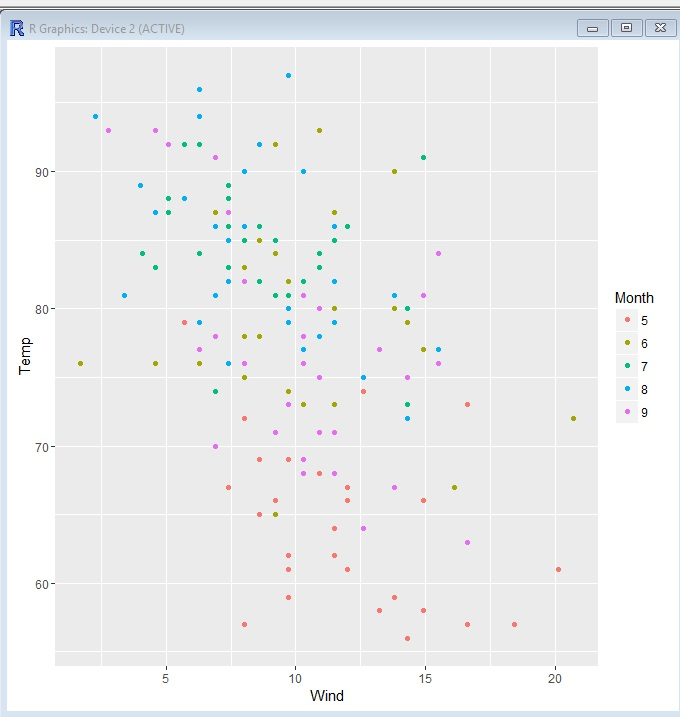`ggplot(data = airQ, aes(x = Wind, y = Temp, color = Month, size = Ozone)) + geom_point()`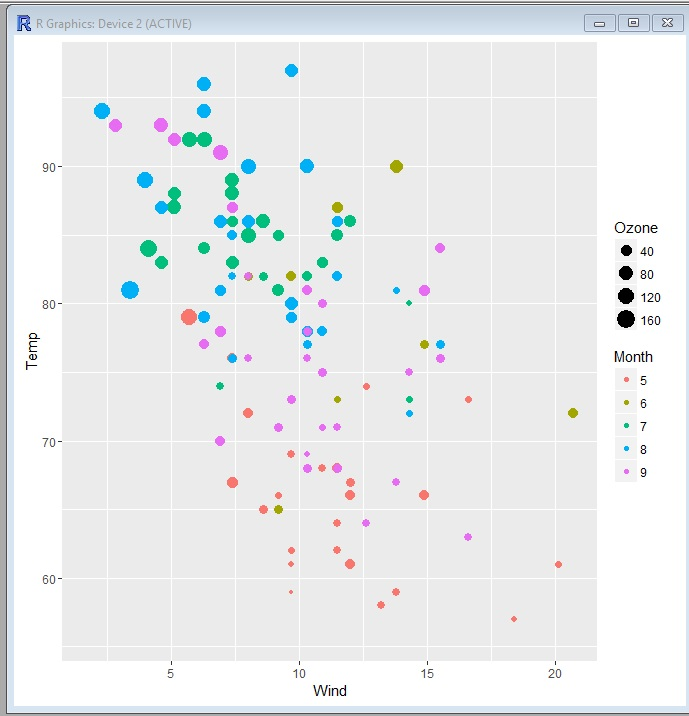## The Code

```library(ggplot2)

#-- assign data to a new variable
airQ <- airquality

#-- look at structure
str(airquality)

#-- set month as a factor
airQ\$Month <- factor(airQ\$Month)
str(airQ)

#-- plot wind vs temp - scatter plot
ggplot(data = airQ, aes(x = Wind, y = Temp)) + geom_point()

#-- plot add factor (Month) as a color
ggplot(data = airQ, aes(x = Wind, y = Temp, color = Month)) + geom_point()

#-- set Ozone as a size element
ggplot(data = airQ, aes(x = Wind, y = Temp, color = Month, size = Ozone)) + geom_point()```

# R: Intro to qplot()

qplot() stands for Quick Plot. It is an easy to use plotting tool for R. In order to use qplot(), you need to have ggplot2 installed. To see if you have it, just type the following:

`library(ggplot2)`

If you get an error, you will need to install the package. To install package:

```install.packages("ggplot2")

library(ggplot2)```

Okay, now let’s get some data. R comes will a great collection of data sets you can work with. You can search Google for R data sets. A great source is also the following webpage: https://stat.ethz.ch/R-manual/R-devel/library/datasets/html/00Index.html

I have decided for this exercise to use the ChickWeight data set listed below.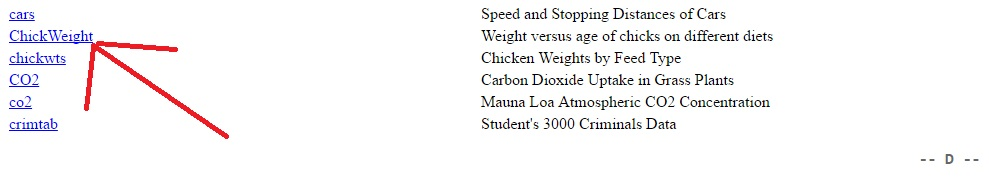To access the dataset in R, simply use the name.

As you can see, the data set contains 4 columns, weight, Time, Chick, Diet

`head(ChickWeight)`Using qplot(), we can get right down to plotting. The basic syntax is: qplot(data, x value)

`qplot(data=ChickWeight, x = weight)`

Notice qplot() automatically labeled our axis. It even created automatic bins for us.`qplot(data=ChickWeight, x = weight, y = Time)`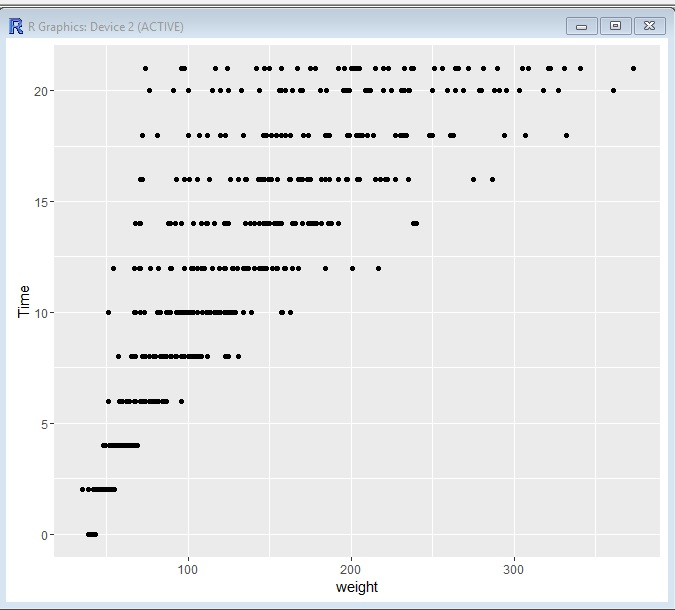Let’s add Diets to the mix now.

`qplot(data=ChickWeight, x= weight, y = Time, color =Diet)`

What jumps out at me right away is the fact the Diet 1 always seems to trail in weight, while Diet 4 seems to lead in weight gain in the earlier weeks, but it is overtaken by 2 and 3 starting around week 12.Now let us add a size element to our graph

`qplot(data=ChickWeight, x= Time, y = Chick,size=weight, color =Diet)`

Now we have Chick’s on the y axis, Time on the X, the size of the dot correlates to weight and the color is diet.

The first thing I notice now is that our data is skewed. We have a lot more chicks on diet 1 than on the other 3 diets. That could effect how we read this data.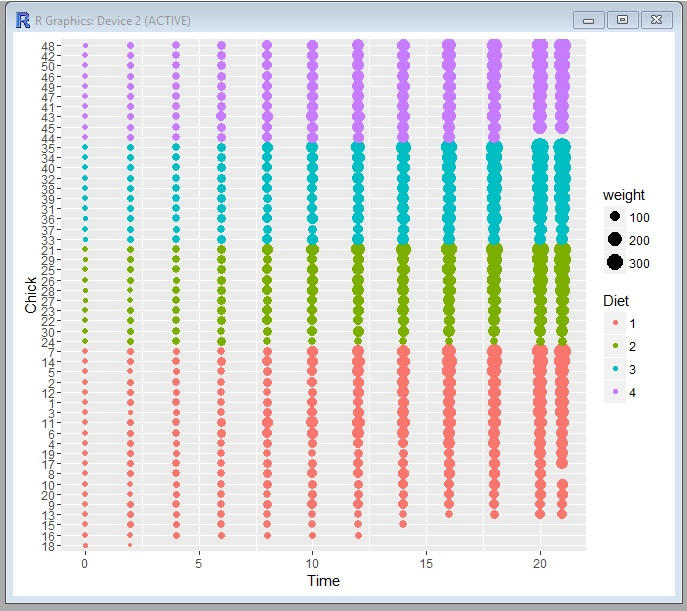And finally, one more just for fun.

`qplot(data=ChickWeight, x= weight, y = Chick, color =Diet)`